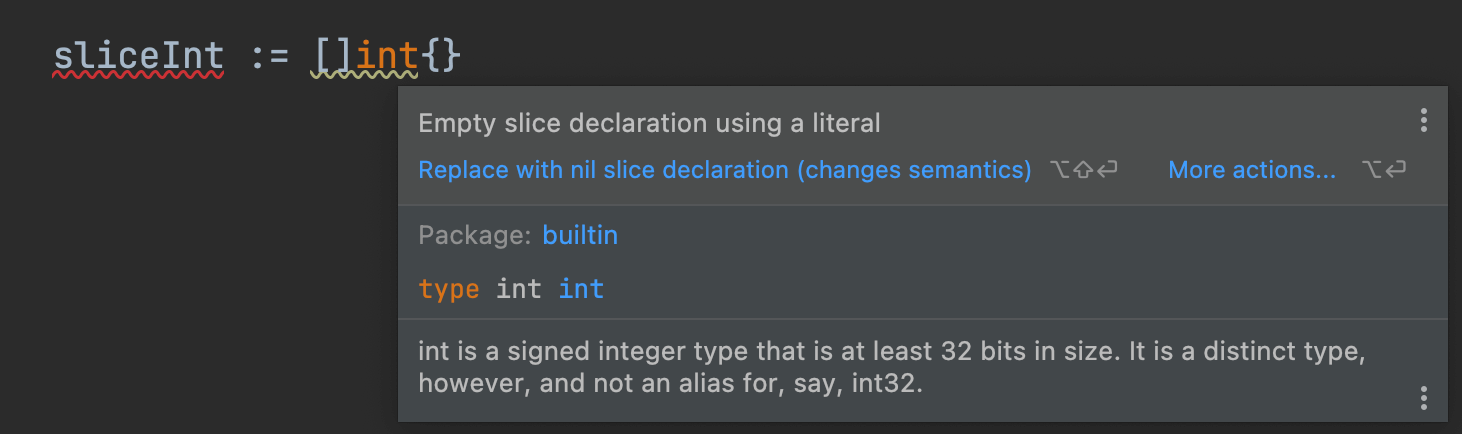# Go - 指针声明

2022-01-09 · xiejiahe

``````var n int
// 或者
n := 0
``````

``````var sliceN1 []int // 结果为 nil

sliceN2 := []int{} // 结果为 []
``````

``````When storage is allocated for a variable, either through a declaration or a call of new, or when a new value is created, either through a composite literal or a call of make, and no explicit initialization is provided, the variable or value is given a default value. Each element of such a variable or value is set to the zero value for its type: false for booleans, 0 for numeric types, “” for strings, and nil for pointers, functions, interfaces, slices, channels, and maps. This initialization is done recursively, so for instance each element of an array of structs will have its fields zeroed if no value is specified.
``````

``````var n []string
r, _ := json.Marshal(n)
fmt.Println(string(r)) // "null"

n := []string{}
r, _ := json.Marshal(n)
fmt.Println(string(r)) // "[]"
````````````var n []int = make([]int, 0) // 全局
sliceN := make([]int, 0) // 函数内
``````

Golang